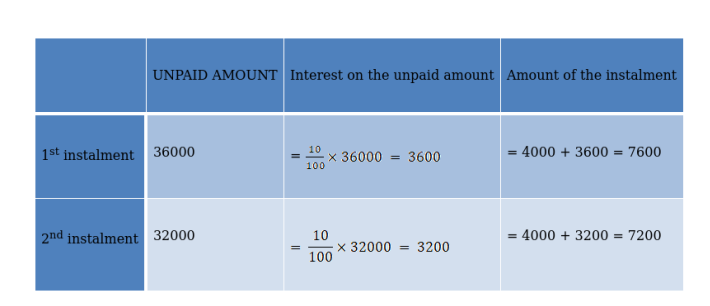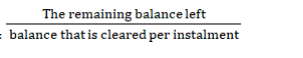# A farmer buys a used for ₹180000.

Question:

A farmer buys a used for ₹180000. He pays ₹90000 in cash and agrees to pay the balance in annual instalments of ₹9000 plus 12% interest on the unpaid amount. How much did the tractor cost him?

Solution:

Given: -

The amount that is to be paid to buy a tractor $=₹ 180000$.

An amount that he paid by cash $=₹ 90000$.

Remaining balance $=₹ 90000$

Annual instalment $=₹ 9000+$ interest @12\% on unpaid amount.Thus, our instalments are 19800, 18720, 17640……

Total number of instalments =$=\frac{90000}{9000}$

= 10

So our instalments are 19800, 18720, 17640 ... upto 10 terms.

All our instalments are in A.P with a common difference d.

Here

First term(a) = 19800

Common difference = d = 18720 - 19800

d = - 1080

Number of terms is 10

Sum of all instalments $=s_{n}=\frac{n}{2}\{2 \times a+(n-1) \times d\}$

$=\frac{10}{2}\{2 \times 19800+(10-1) \times(-1080)\}$

= 149400

Hence,

The total cost of the scooter = amount that is paid earlier + amount paid in 10 instalments.

= 90000 + 149400

∴The total cost paid by the farmer = ₹239400